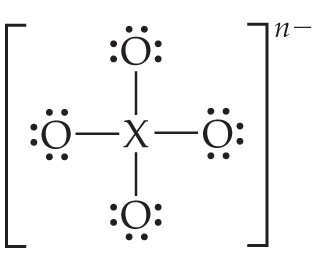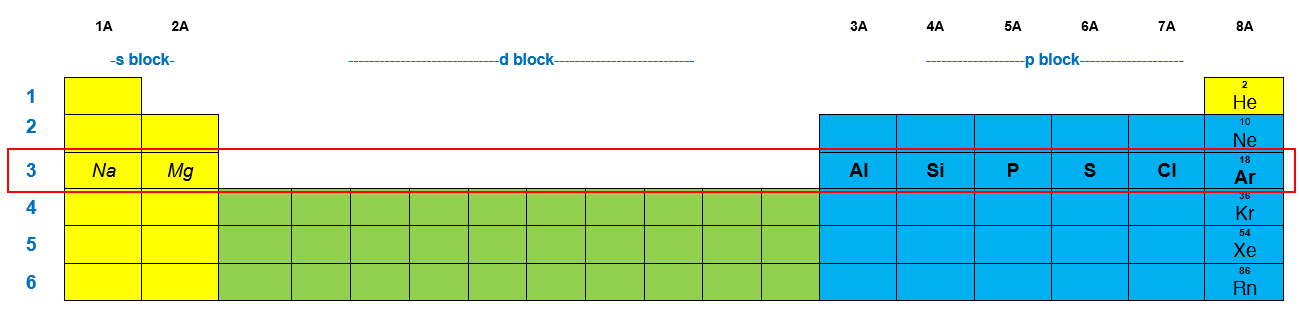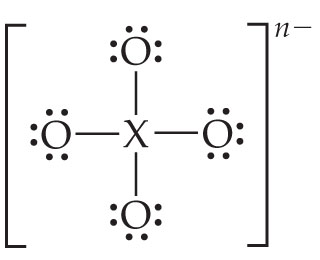# Problem: Consider the Lewis structure for the polyatomic oxyanion shown here, where X is an element from the third period (Na-Ar). By changing the overall charge, n, from 1- to 2- to 3- we get three different polyatomic ions.For each of these ions identify the central atom, X. Arrange your answers in order increasing n.

###### FREE Expert Solution

We’re asked to identify the central atom, X for each of these ions where n = 1-, 2- and 3- based on the given Lewis structure of the polyatomic oxyanion:We know that the element, X comes from the third period (Na to Ar) and when it is bonded to 4 O's it forms a polyatomic oxyanionRecall that a polyatomic oxyanion is a molecule consisting of a nonmetal bonded to more than one Oxygen atoms (covalent bond).  This means that X cannot be a metal (metal + nonmetal = ionic bond)

To identify the atom, X for each charge n, we need to do these steps:

Step 1: Determine the number of valence electrons for the central atom, X for each charge, n

Step 2: Determine Group Number and corresponding element X for each polyatomic oxyanion

From the given Lewis structure, there are a total of [4 x 6] + 4 (bonds) = 32 valence e

**The line (—) represents a "bond" where 2 electrons are shared between two atoms

**Each dot represents 1 electron (for every O atom there are 6 dots = 6 e)

94% (13 ratings)###### Problem Details
Consider the Lewis structure for the polyatomic oxyanion shown here, where X is an element from the third period (Na-Ar). By changing the overall charge, n, from 1- to 2- to 3- we get three different polyatomic ions.For each of these ions identify the central atom, X. Arrange your answers in order increasing n.